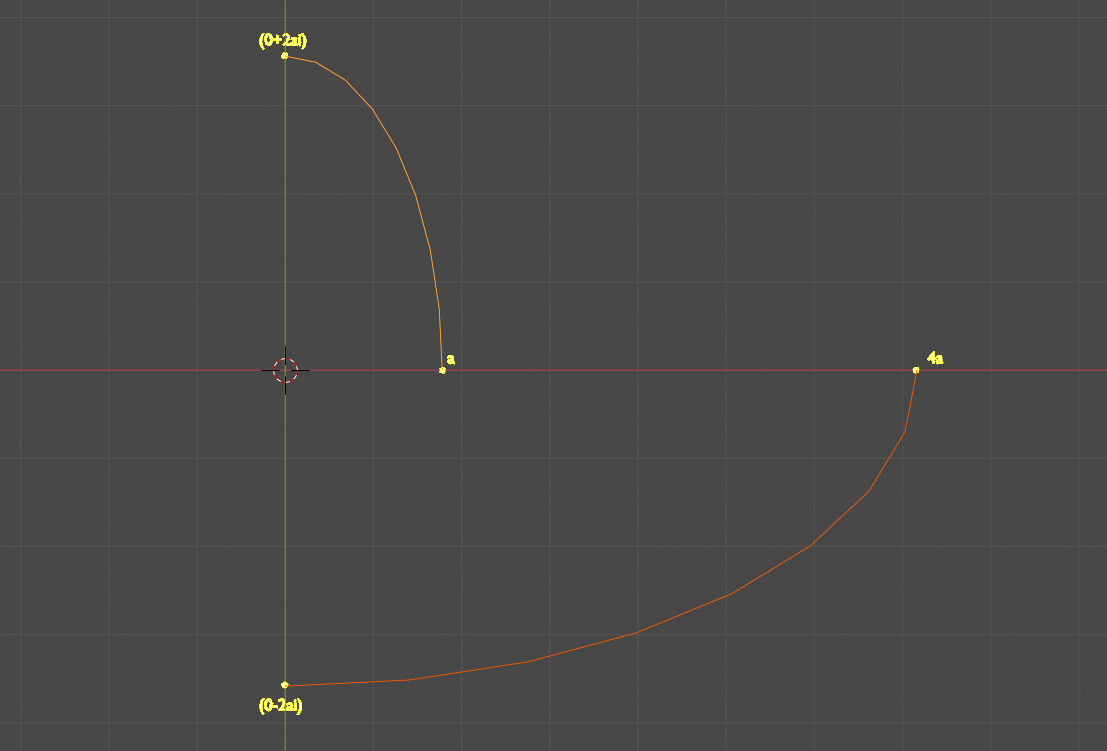0 replies · posted

## Quaternions demystified, intermezzo

Suppose we want to multiply  the real number a by, let’s say 9.

That’s easy, right? 9 * a = 9a. Done!

Now let’s try that again, this time using complex numbers (we just learned about them and are awestruck by what they can do and we want to use them all the time…).

( A complex number is a 2 dimensional number, with a ‘real’ part and an ‘imaginary’ part; notation: a+ bi, a and b are real numbers. I am not going into that, you can Google complex numbers, they are well explained.)

Let’s try this: multiply a by (0 + 9i). Result: (0 + 9ai).

Well, partly a success. The ‘magnitude’ of a is now correct, but the number completely disappeared from the real world; it rotated into the imaginary world. In order to get it back into reality, we will multiply the result by the so-called complex conjugate of (0 + 9i) (again, the name is the only difficult part): (0 – 9i).

Now we get 81 as a result (i * i = -1, so i * -i = 1). We’re back into the real world, but now we have multiplied by 9 twice! Luckily there is an easy solution for this, called the square root.

So instead of 9i, we will use the square root of 9 times i, so: 3i.

The total formula we get is: 9 * a = (0 + 3i) * a * (0 - 3i) = 9a.

Hurray!

(The numbers in the picture are different for scale reasons, principle is the same)Notice that both times we multiplied by a complex number, we rotated in the same direction!